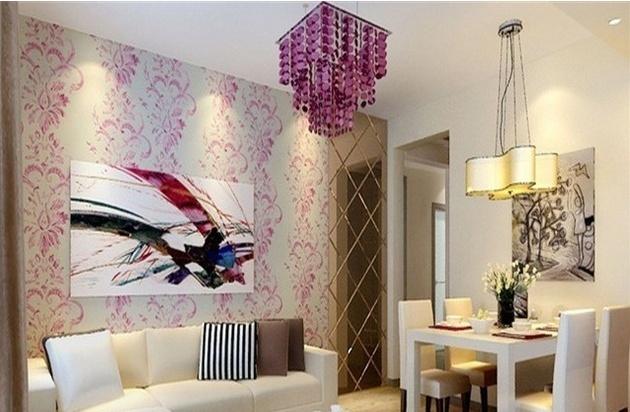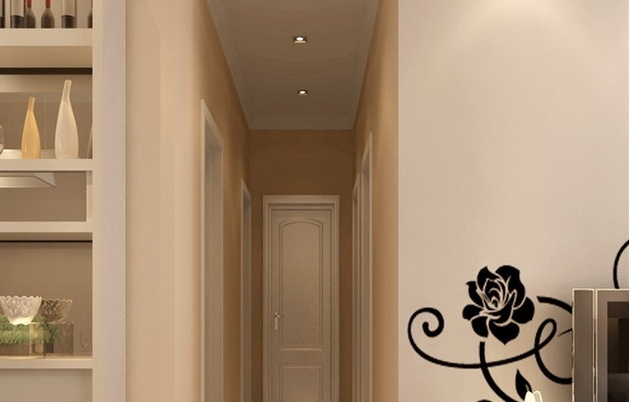|

# 楼房简装装修预算 简装房收房要点楼房简装装修预算 简装房收房要点

1、卫生间：最重要是要做好防水工程，不然万一渗漏楼下投诉就很麻烦。材料主要分为瓷砖及人工，以及浴具的成本，特别强调一下的是软管角阀地漏这些小东西，品牌的和咋牌的价格相差不大但是质量千差万别，我相信没有房东愿意经常接到租客电话，说今天这里漏水，明天那里渗水。

所以这些小东西尽量都选择品牌。比如杭特。软管也就8-10元一根，角阀17-20一只。千万不要在公共卫生间里装马桶，一定要用蹲坑，经济实惠更受欢迎。吊顶不是必须的，可做可不做。单个卫生间的全部费用加起来差不多要3500以上。

2、地板：出租房地板当然选择强化地板，强化地板主要是由4块组成，最下面的是防潮层，然后是基层(每种地板的材料不一样)，、上面的是装饰层，再就是耐磨层。强化地板现在市场上一般是0.8cm和1.2cm厚的，对于出租房来说，选择0.8的就足够了，从环保上来说，也要0.8的更环保，因为更薄的地板单位面积里所用的胶要少。

现在市场上的强化地板良莠不齐，选个中等价位的就差不多，便宜的有10几块的，贵的有大几百一平方的，一般选择40元/方左右的即可。

另外，现在市场上的地板根本就不存在防水的，如果有商家跟你说他地板上防水，那你得小心了。不要忘了还有地板辅材和损耗指标，如地脚线，说实话其实就是完全为装饰而做的，选个一般的即可，地脚线，铜条等辅材大概5块钱/平方。铺设地板的正常损耗在5-6%，根据师傅的手艺而定，手艺差的损耗肯定大。楼房简装装修预算 简装房收房要点

3、门：现在市场上有3种比较常见的门，第1个是免漆套装门，第2个是烤漆套装门(此类门一般用于家装，比较贵)，第3种就是买材料让木工师傅现场做，这类门的话有个缺点就是要油漆，有油漆必然会释放甲醛，必然不环保，需要晾上一段时间，但是对于出租房来说，每闲置一天，就等于少了一天的租金收入。

所以一般比较推荐免漆套装门，此类门安装方便，且价格不高，质量比较好的在1000左右，对于出租房来说，选个耐用的就行，差不多总造价要700左右/扇(含锁，门吸、合页，及安装人工费)。注意卫生间如果面积比较小，水容易溅到门上，推荐还是用能防水的塑钢门。

门锁的选择也有讲究，房间门锁不推荐买最便宜的球形锁，这种锁寿命短很容易损坏，维修成本高，至少要买有2年质保的中档锁。卫生间锁可以考虑用不带钥匙的球形锁，记得再买个插销让师傅安在门内侧，以防球形锁坏了，这时还能把锁就当把手用。

4、隔断：每增加一个房间，装修成本大概增加5000左右，但每年至少可以给房东增加6-7000元的租金收入，所以在不影响房屋品质和安全的前提下，在保证每个房间都有晾晒空间的前提下，可以考虑把厨房，客厅，或餐厅隔出来增加1-2个居住的房间，这样能显著增加租金收入。

现在目前市场上采用的隔墙材料要么是轻钢龙骨+石膏板，要么是轻质砖砌墙，两个方案成本差不多，都在70元/方左右，但轻质砖的消防和隔音方面更好些，会让租客的居住更有安全感!

5、厨房：按常规的做法，地砖，墙砖加橱柜就可以了。需要注意的是燃气灶的安全性，最好去燃气公司买个最便宜的，万一出事有他们兜着。另外天然气的管道接头和燃气灶之间的连接软管最好使用80元一根的长命的金属管，橡胶管一般1年寿命，天然气要出事都是大事，一定要谨慎!楼房简装装修预算 简装房收房要点

6.家俱家电：家具的重点是床，200元以下的床建议不要买，绝对不超过一年的寿命，如果碰到租客比较胖的话，估计半年不到就over了。所以，床一定要选择质量比较好，比较坚固的。席梦思有太多种，一定要买厂家给质保最少1年以上的，那种没有售后的千万不要买，有可能睡几个晚上就塌陷。

桌子椅子也是要求结实耐用。另外一个重点就是家电，特别是热水器，洗衣机和空调这几样必配的，建议买全新的二线品牌，不要买二手，而且一定要买正规渠道并且有3年以上售后质保的家电，这样您就省事多了

7、软装：软装可以迅速提升装修品位，是简装修中比不可少的部分。简装修的窗帘在100-200元每幅即可，墙壁上可以安装一些小的壁挂塑料花篮，也可使用一些色彩亮丽的壁灯来做些点缀，另外如果主要面对年轻的租客，可以考虑刷几面有活波颜色的颜色墙来让整个房子显得更生动。楼房简装装修预算 简装房收房要点

简装房收房要点

业主购买这样的“简装房”，基本上厨房、卫生间就不用装修了如果业主想要改造一下也是可以最多购置一些高品质的产品，但是水电路就不用进行改造了免得造成不必要的麻烦从新花钱。大家购买简装房就是图省事图省钱，能不动的就不要去动。而简装房收房的重点就是检查它装修的效果是否符合标准，建议业主要仔细查看水电路，一面日后使用起来频繁出现故障，最好询问好物业这些装修的信息，是什么产品以及有没有售后，出现故障该联系谁。

`声明：本文由入驻焦点开放平台的作者撰写，除焦点官方账号外，观点仅代表作者本人，不代表焦点立场错误信息举报电话： 400-099-0099，邮箱：jubao@vip.sohu.com，或点此进行意见反馈，或点此进行举报投诉。`A B C D E F G H J K L M N P Q R S T W X Y Z
A - B - C - D - E
• A
• 鞍山
• 安庆
• 安阳
• 安顺
• 安康
• 澳门
• B
• 北京
• 保定
• 包头
• 巴彦淖尔
• 本溪
• 蚌埠
• 亳州
• 滨州
• 北海
• 百色
• 巴中
• 毕节
• 保山
• 宝鸡
• 白银
• 巴州
• C
• 承德
• 沧州
• 长治
• 赤峰
• 朝阳
• 长春
• 常州
• 滁州
• 池州
• 长沙
• 常德
• 郴州
• 潮州
• 崇左
• 重庆
• 成都
• 楚雄
• 昌都
• 慈溪
• 常熟
• D
• 大同
• 大连
• 丹东
• 大庆
• 东营
• 德州
• 东莞
• 德阳
• 达州
• 大理
• 德宏
• 定西
• 儋州
• 东平
• E
• 鄂尔多斯
• 鄂州
• 恩施
F - G - H - I - J
• F
• 抚顺
• 阜新
• 阜阳
• 福州
• 抚州
• 佛山
• 防城港
• G
• 赣州
• 广州
• 桂林
• 贵港
• 广元
• 广安
• 贵阳
• 固原
• H
• 邯郸
• 衡水
• 呼和浩特
• 呼伦贝尔
• 葫芦岛
• 哈尔滨
• 黑河
• 淮安
• 杭州
• 湖州
• 合肥
• 淮南
• 淮北
• 黄山
• 菏泽
• 鹤壁
• 黄石
• 黄冈
• 衡阳
• 怀化
• 惠州
• 河源
• 贺州
• 河池
• 海口
• 红河
• 汉中
• 海东
• 怀来
• I
• J
• 晋中
• 锦州
• 吉林
• 鸡西
• 佳木斯
• 嘉兴
• 金华
• 景德镇
• 九江
• 吉安
• 济南
• 济宁
• 焦作
• 荆门
• 荆州
• 江门
• 揭阳
• 金昌
• 酒泉
• 嘉峪关
K - L - M - N - P
• K
• 开封
• 昆明
• 昆山
• L
• 廊坊
• 临汾
• 辽阳
• 连云港
• 丽水
• 六安
• 龙岩
• 莱芜
• 临沂
• 聊城
• 洛阳
• 漯河
• 娄底
• 柳州
• 来宾
• 泸州
• 乐山
• 六盘水
• 丽江
• 临沧
• 拉萨
• 林芝
• 兰州
• 陇南
• M
• 牡丹江
• 马鞍山
• 茂名
• 梅州
• 绵阳
• 眉山
• N
• 南京
• 南通
• 宁波
• 南平
• 宁德
• 南昌
• 南阳
• 南宁
• 内江
• 南充
• P
• 盘锦
• 莆田
• 平顶山
• 濮阳
• 攀枝花
• 普洱
• 平凉
Q - R - S - T - W
• Q
• 秦皇岛
• 齐齐哈尔
• 衢州
• 泉州
• 青岛
• 清远
• 钦州
• 黔南
• 曲靖
• 庆阳
• R
• 日照
• 日喀则
• S
• 石家庄
• 沈阳
• 双鸭山
• 绥化
• 上海
• 苏州
• 宿迁
• 绍兴
• 宿州
• 三明
• 上饶
• 三门峡
• 商丘
• 十堰
• 随州
• 邵阳
• 韶关
• 深圳
• 汕头
• 汕尾
• 三亚
• 三沙
• 遂宁
• 山南
• 商洛
• 石嘴山
• T
• 天津
• 唐山
• 太原
• 通辽
• 铁岭
• 泰州
• 台州
• 铜陵
• 泰安
• 铜仁
• 铜川
• 天水
• 天门
• W
• 乌海
• 乌兰察布
• 无锡
• 温州
• 芜湖
• 潍坊
• 威海
• 武汉
• 梧州
• 渭南
• 武威
• 吴忠
• 乌鲁木齐
X - Y - Z
• X
• 邢台
• 徐州
• 宣城
• 厦门
• 新乡
• 许昌
• 信阳
• 襄阳
• 孝感
• 咸宁
• 湘潭
• 湘西
• 西双版纳
• 西安
• 咸阳
• 西宁
• 仙桃
• 西昌
• Y
• 运城
• 营口
• 盐城
• 扬州
• 鹰潭
• 宜春
• 烟台
• 宜昌
• 岳阳
• 益阳
• 永州
• 阳江
• 云浮
• 玉林
• 宜宾
• 雅安
• 玉溪
• 延安
• 榆林
• 银川
• Z
• 张家口
• 镇江
• 舟山
• 漳州
• 淄博
• 枣庄
• 郑州
• 周口
• 驻马店
• 株洲
• 张家界
• 珠海
• 湛江
• 肇庆
• 中山
• 自贡
• 资阳
• 遵义
• 昭通
• 张掖
• 中卫

1室1厅1厨1卫1阳台

1
2
3
4
5

0
1
2

1

1

0
1
2
3报名成功，资料已提交审核A B C D E F G H J K L M N P Q R S T W X Y Z
A - B - C - D - E
• A
• 鞍山
• 安庆
• 安阳
• 安顺
• 安康
• 澳门
• B
• 北京
• 保定
• 包头
• 巴彦淖尔
• 本溪
• 蚌埠
• 亳州
• 滨州
• 北海
• 百色
• 巴中
• 毕节
• 保山
• 宝鸡
• 白银
• 巴州
• C
• 承德
• 沧州
• 长治
• 赤峰
• 朝阳
• 长春
• 常州
• 滁州
• 池州
• 长沙
• 常德
• 郴州
• 潮州
• 崇左
• 重庆
• 成都
• 楚雄
• 昌都
• 慈溪
• 常熟
• D
• 大同
• 大连
• 丹东
• 大庆
• 东营
• 德州
• 东莞
• 德阳
• 达州
• 大理
• 德宏
• 定西
• 儋州
• 东平
• E
• 鄂尔多斯
• 鄂州
• 恩施
F - G - H - I - J
• F
• 抚顺
• 阜新
• 阜阳
• 福州
• 抚州
• 佛山
• 防城港
• G
• 赣州
• 广州
• 桂林
• 贵港
• 广元
• 广安
• 贵阳
• 固原
• H
• 邯郸
• 衡水
• 呼和浩特
• 呼伦贝尔
• 葫芦岛
• 哈尔滨
• 黑河
• 淮安
• 杭州
• 湖州
• 合肥
• 淮南
• 淮北
• 黄山
• 菏泽
• 鹤壁
• 黄石
• 黄冈
• 衡阳
• 怀化
• 惠州
• 河源
• 贺州
• 河池
• 海口
• 红河
• 汉中
• 海东
• 怀来
• I
• J
• 晋中
• 锦州
• 吉林
• 鸡西
• 佳木斯
• 嘉兴
• 金华
• 景德镇
• 九江
• 吉安
• 济南
• 济宁
• 焦作
• 荆门
• 荆州
• 江门
• 揭阳
• 金昌
• 酒泉
• 嘉峪关
K - L - M - N - P
• K
• 开封
• 昆明
• 昆山
• L
• 廊坊
• 临汾
• 辽阳
• 连云港
• 丽水
• 六安
• 龙岩
• 莱芜
• 临沂
• 聊城
• 洛阳
• 漯河
• 娄底
• 柳州
• 来宾
• 泸州
• 乐山
• 六盘水
• 丽江
• 临沧
• 拉萨
• 林芝
• 兰州
• 陇南
• M
• 牡丹江
• 马鞍山
• 茂名
• 梅州
• 绵阳
• 眉山
• N
• 南京
• 南通
• 宁波
• 南平
• 宁德
• 南昌
• 南阳
• 南宁
• 内江
• 南充
• P
• 盘锦
• 莆田
• 平顶山
• 濮阳
• 攀枝花
• 普洱
• 平凉
Q - R - S - T - W
• Q
• 秦皇岛
• 齐齐哈尔
• 衢州
• 泉州
• 青岛
• 清远
• 钦州
• 黔南
• 曲靖
• 庆阳
• R
• 日照
• 日喀则
• S
• 石家庄
• 沈阳
• 双鸭山
• 绥化
• 上海
• 苏州
• 宿迁
• 绍兴
• 宿州
• 三明
• 上饶
• 三门峡
• 商丘
• 十堰
• 随州
• 邵阳
• 韶关
• 深圳
• 汕头
• 汕尾
• 三亚
• 三沙
• 遂宁
• 山南
• 商洛
• 石嘴山
• T
• 天津
• 唐山
• 太原
• 通辽
• 铁岭
• 泰州
• 台州
• 铜陵
• 泰安
• 铜仁
• 铜川
• 天水
• 天门
• W
• 乌海
• 乌兰察布
• 无锡
• 温州
• 芜湖
• 潍坊
• 威海
• 武汉
• 梧州
• 渭南
• 武威
• 吴忠
• 乌鲁木齐
X - Y - Z
• X
• 邢台
• 徐州
• 宣城
• 厦门
• 新乡
• 许昌
• 信阳
• 襄阳
• 孝感
• 咸宁
• 湘潭
• 湘西
• 西双版纳
• 西安
• 咸阳
• 西宁
• 仙桃
• 西昌
• Y
• 运城
• 营口
• 盐城
• 扬州
• 鹰潭
• 宜春
• 烟台
• 宜昌
• 岳阳
• 益阳
• 永州
• 阳江
• 云浮
• 玉林
• 宜宾
• 雅安
• 玉溪
• 延安
• 榆林
• 银川
• Z
• 张家口
• 镇江
• 舟山
• 漳州
• 淄博
• 枣庄
• 郑州
• 周口
• 驻马店
• 株洲
• 张家界
• 珠海
• 湛江
• 肇庆
• 中山
• 自贡
• 资阳
• 遵义
• 昭通
• 张掖
• 中卫• 手机• 分享
• 设计
免费设计
• 计算器
装修计算器
• 入驻
合作入驻
• 联系
联系我们
• 置顶
返回顶部